• Call Now

1800-102-2727•

# Trapezium: Definition, Types, Properties, Formula, Practice Problems and FAQs

A trapezium is a quadrilateral having one set of parallel sides in a two-dimensional space. Because all of its inner angles are less than 180° and its vertices point outward, it is a convex quadrilateral. It has four vertices and four sides. The two sides are non-parallel and are referred to as legs, whereas the parallel sides are known as bases. In this article, we will learn about trapezium, its characteristics and other aspects with the help of some practice problems.

• Definition of Trapezium
• Types of Trapezium
• Perimeter of Trapezium
• Area of Trapezium
• Derivation of the Formula of Area of Trapezium
• Midpoint Theorem on Trapezium
• Practice Problems
• FAQs

## Definition of Trapezium

A quadrilateral with only one set of parallel opposite sides is a trapezium. The bases and legs of a trapezium are referred to as parallel and non-parallel sides, respectively. It is also known as a trapezoid.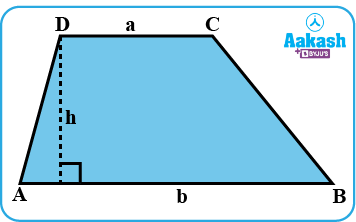Trapezium

In the above diagram, ABCD is the trapezium, with parallel bases a and b and h is the height of the trapezium.

### Some Important Terms to Remember

• Bases: The parallel sides (a and b) of the trapezium are called the bases of the trapezium.
• Legs or lateral sides: The legs are the non-parallel sides of the trapezium.
• Altitude or height: The altitude or height (h) of the trapezium is the perpendicular distance between its two bases.

## Types of Trapezium

There are three types of trapezium:

1. Scalene Trapezium
2. Isosceles Trapezium
3. Right Trapezium

### Scalene Trapezium

A trapezium whose angles and sides are of different measure is known as a scalene trapezium.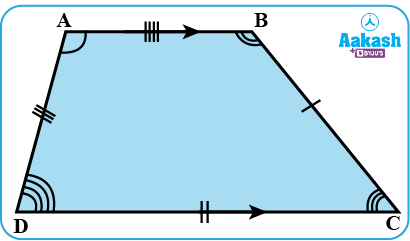Scalene Trapezium

### Isosceles Trapezium

The trapezium whose base angles are congruent and whose non-parallel sides or legs are of equal length is called an Isosceles trapezium.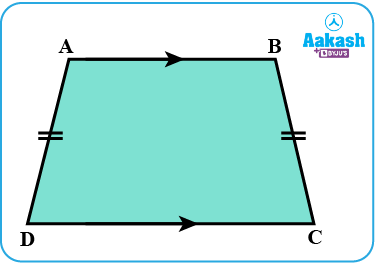Isosceles Trapezium

### Right Trapezium

A trapezium is said to be a right trapezium if it has at least one right angle.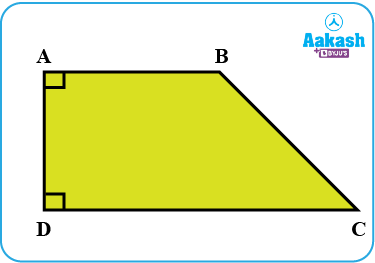Right Trapezium

## Perimeter of Trapezium

The sum of all the 4 side lengths of a trapezium is the Perimeter of a trapezium. It is denoted by P and measured in units like m, cm, mm, etc.

The formula to calculate the perimeter of the trapezium, P=a+b+c+d units

Where, a, b, c and d are the side lengths of the trapezium, as shown in the figure below.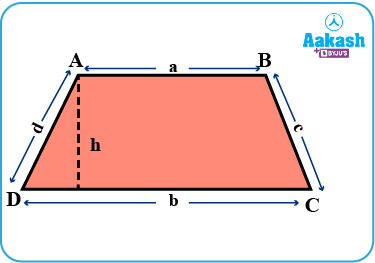Perimeter of Trapezium

## Area of Trapezium

The area of the trapezium is the space covered by a trapezium on a two-dimensional plane. It is denoted by A and measured in square units like m2, cm2, mm2, etc. The area of trapezium can be calculated by taking the average of parallel sides and multiplying by its height.

The formula to calculate the area of a trapezium is,

Where, a and b are the lengths of the parallel sides or bases of the trapezium, and h is the height or altitude of the trapeziumArea of Trapezium

## Derivation of the Formula of Area of Trapezium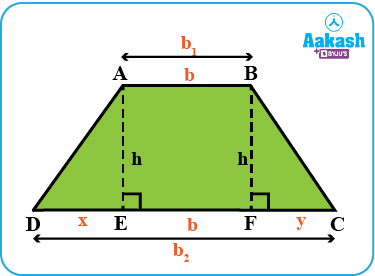From the above diagram, we can see that given trapezium comprises of Δ AED, Δ BFC and rectangle ABFE.

In Δ AED, the base is x and height is h.

In Δ BFC, the base is y and and height is h.

In rectangle ABFE, length is b and width is h.

Also,

${b}_{1}=b$

${b}_{2}=x+b+y$

Now, the area of trapezium is equal to the sum of the area of two triangles and the area of the rectangle.

Since, ${b}_{1}=b$

${b}_{2}=x+y+b$

Hence, the area of trapezium

## Midpoint Theorem on Trapezium

Theorem: The median of a trapezium is parallel to the bases and half the sum of the length of the bases.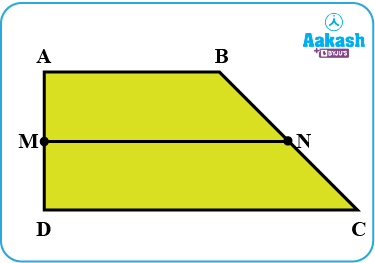Midpoint Trapezium

Given: ABCD is a trapezium and MN is the median.

To prove: MN || AB, MN || DC and MN=12(AB+DC)

Proof:

Draw a line DN extending from D until it intersects with the extension of AB, at P.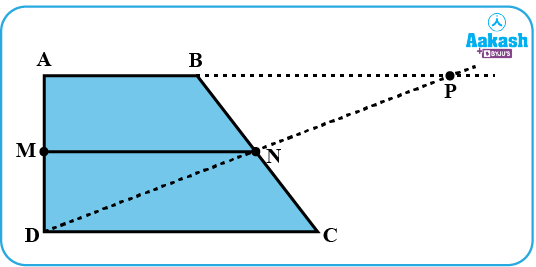N is the midpoint of BC and M is the midpoint of AD [∵ MN is the line joining midpoints]

In DCN and PBN

Then, ∠ DCN = PBN [Alternate interior angles]

∠ DNC = PNB [Vertically opposite angles]

BN=NC [N is the midpoint]

Now, we get

DCN ≅ PBN [ASA Congruence Rule]

DN = NP ; DC =BP [CPCT]

Now, in DAP

N is the midpoint of DP and M is the midpoint of AD

Hence, by mid-point theorem

From above, we get

MN || AP and MN=12AP

If MN || APMN || AB

Also, MN || CD [∵ AB || CD]

Now,

$MN=\frac{1}{2}AP$(Midpoint theorem of the triangle)

$MN=\frac{1}{2}\left(AB+BP\right)$

$MN=\frac{1}{2}\left(AB+DC\right)$ [∵ BP=DC]

Hence, proved.

## Properties of Trapezium

1. The Sum of angles between the bases and one of the legs is equal to 180.
2. The mean of both parallel sides is the median length of a trapezium also called a midsegment.
3. In an isosceles trapezium, base angles are equal.

## Practice Problems

Question 1: Find the perimeter of the trapezium whose side lengths are6 cm, 9 cm, 12 cm and 10 cm.

Solution:

We know that, the perimeter of the trapezium is the sum of its side lengths.

Hence, Perimeter =P=6+9+12+10=37 cm

Question 2: Find the area of the trapezium whose bases are of 6 cm and 12 cm in length and the distance between the parallel bases is 4 cm.

Solution: Let a and b be 6 cm and 12 cm, respectively and h be the distance between the two parallel bases i.e., 4 cm

We know, Area of the trapezium

Hence,

Question 3: Find the height of the trapezium whose area is 120 cm2 and the sum of the parallel sides is 12 cm.

Solution: We have, Area =A=120 cm2 and Sum of parallel sides =(a+b)=12 cm

We know, Area of the trapezium

Hence, the height of the trapezium is 20 cm.

Question 4: Find the length of the midsegment of the trapezium if its parallel sides are 8 cm and 19 cm.

Solution: Let a and b be sides of trapezium, given 8 cm and 19 cm, respectively.

We know, Length of the midsegment $\left(L\right)=\frac{1}{2}\left(a+b\right)$

Hence, the length of the midsegment is 13.5 cm.

Question 5: Find all the missing angles in isosceles trapezium given below.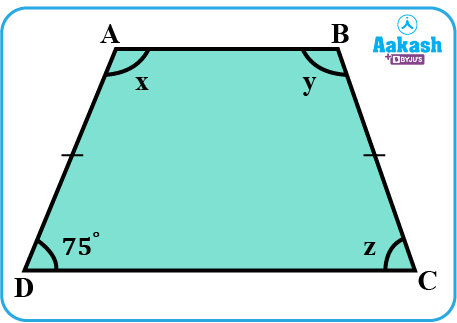Solution: We have, D= 75°.

We know that the base angles of an isosceles trapezium are equal.

z=D= 75°

Also, we know that the sum of angles between the bases and one of the legs is equal to 180.

Therefore,

$x=180°-75°=105°$

Also,

$y=180°-75°=105°$

Hence, the other angles of the given trapezium are 75°, 105°,75° and 105°, respectively.

## FAQs

1. Which type of trapezium has equal base angles?
Isosceles trapezium is the only trapezium that has equal base angles.

2. Can a trapezium have equal diagonals? If yes, state the condition.
Yes, the isosceles trapezium has equal diagonals because it has a pair of equal sides (legs).

3. Do the diagonals of a trapezium bisect each other?
No, the diagonals of the trapezium do not bisect each other.

Yes, the trapezium is a quadrilateral with 4 sides, 4 angles and 4 vertices. The sum of the interior angles is 360°.

5. Can we say a parallelogram is a trapezium?
No, a parallelogram is not a trapezium. Because in a parallelogram, both the pair of opposite sides are parallel. Whereas in trapezium only one pair of opposite sides is parallel.Talk to our expert
Resend OTP Timer =
By submitting up, I agree to receive all the Whatsapp communication on my registered number and Aakash terms and conditions and privacy policy